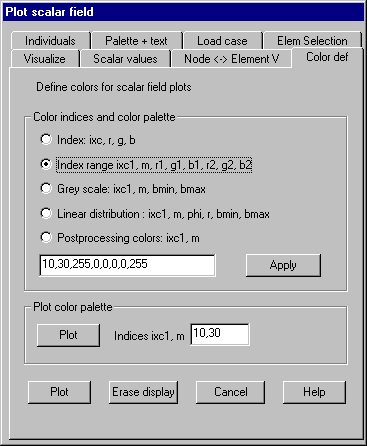Color definition: Define color values for color indices

This property page redefines colors associated to color indices. RGB-values are to be given in the range of 0 – 255.

Following dialog shows the available options:Index: ixc, r, g, b

The color index and associated RGB-values must be given for a single index

Index range: ixc1, m, r1, g1, b1, r2, g2, b2

The smallest color index ixc1 and the number m of indices for which new values should be defined together with RGB-values for the smallest and the largest color index must be given. Color values for all indices between are calculated using linear interpolation.

Grey scale: ixc1, m, bmin, bmax

The smallest color index ixc1 and the number m of color indices together with the brightness value for the smallest and the largest index must be given in %. Indices between are calculated using linear interpolation.

Linear: ixc1, m, phi, bmin, bmax

The smallest color index ixc1, the number of indices m, the color value phi, the smallest brightness bmin and the largest brightness bmax must be given. Color is defined by an angle phi (0-360), a radius r (0-100) and a brightness b (0-100) of a color cone (HLS-system).

Following colors are assigned to angles: 0° = blue, 60° = magenta, 120° = red, 180° = yellow, 240° = green, 300° = cyan, 360° = blue.

Post processing colors: ixc1, m

The smallest color index ixc1 and the number m of following indices must be given. Between these indices a smooth color transition of the colors blue, green, yellow, red and magenta will be created.

Apply

After setting the options this button does the calculation of the colors and updates the color lookup tables.

Plot color palette

This button plots current color settings as a color bar for indices ixc1 until ixc1+m-1.

Button „Plot“ updates the current scalar data plot with changed color settings.